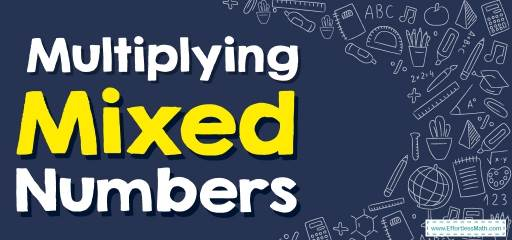# How to Multiply Mixed Numbers? (+FREE Worksheet!)

Is it hard for you to multiply mixed numbers? In this post, you learn to multiply mixed numbers in a few simple steps. So join us!Fractions greater than $$1$$ are usually represented as mixed numbers. In this case, the mixed number consists of an integer part and a standard fraction less than $$1$$. The integer part is the same as the quotient part and the numerator of the fraction is the remainder of the division and the denominator of the fraction will also be the divisor.

Best Math for Grade 5 Resource

## Step-by-step guide to multiplying mixed numbers

In the Multiplication of mixed numbers, their improper fractions can be used instead, and if necessary, the result can be converted back to the form of mixed numbers.

Here’s how to multiply mixed numbers:

• Step 1: Convert all mixed numbers into improper fractions (improper fraction is a fraction in which the top number is bigger than the bottom number): To convert a mixed number to a fraction, you must first multiply the mixed number by the denominator, then add the result to the numerator. Put the resulting number in the case of a new numerator and keep the denominator of the same fraction as the previous one. This way you have converted a mixed number into a fraction. $$a \ \frac{c}{ \color{ blue }{b} }= a \ \color{blue}{+} \ \frac{c}{\color{ blue }{b}}=\frac{a \color{ blue }{b} \ \color{blue}{+} \ c}{ \color{ blue }{b} }$$
• Step 2: multiply these two improper fractions: To multiply fractions, we multiply the first fraction by the second fraction
• Step 3: Convert the answer to a mixed number: To convert a fraction larger than one to a mixed number, you must divide the numerator by the denominator. After division, the obtained quotient is the same integer in the mixed number and the remainder of the division is the numerator of the fraction. The denominator is also the denominator of the initial fraction in the mixed number.

### Multiplying Mixed Numbers – Example 1:

Multiply mixed numbers. $$2 \ \frac{1}{4} \ \times \ 3 \ \frac{1}{2}=$$

Solution:

Converting mixed numbers to fractions, $$2 \ \frac{1}{4}=\frac{9}{4}$$ and $$3 \ \frac{1}{2}=\frac{7}{2}$$.
$$\frac{9}{4} \ \times \ \frac{7}{2}$$,

Applying the fractions rule for multiplication, $$\frac{9}{4} \ \times \ \frac{7}{2}=$$ $$\frac{9 \ \times \ 7}{4 \ \times \ 2}=\frac{63}{8}=7 \ \frac{7}{8}$$

### Multiplying Mixed Numbers – Example 2:

Multiply mixed numbers. $$5 \ \frac{2}{3} \ \times \ 3 \ \frac{3}{4}=$$

Solution:

Converting mixed numbers to fractions, $$5 \ \frac{2}{3}=\frac{17}{3}$$ and $$3 \ \frac{3}{4}=\frac{15}{4}$$.
$$\frac{17}{3} \ \times \ \frac{15}{4}$$,

Applying the fractions rule for multiplication, $$\frac{17}{3} \ \times \ \frac{15}{4}=$$ $$\frac{17 \ \times \ 15}{3 \ \times \ 4}=\frac{255}{12}=\frac {255÷ 3} {12÷ 3} =\frac {85} {4}= 21 \ \frac{1}{4}$$

The Best Math Books for Elementary Students

### Multiplying Mixed Numbers – Example 3:

Multiply mixed numbers. $$3 \ \frac{2}{3} \ \times \ 2 \ \frac{1}{2}=$$

Solution:

Converting mixed numbers to fractions, $$3 \ \frac{2}{3}=\frac{11}{3}$$ and $$2 \ \frac{1}{2}=\frac{5}{2}$$.
$$\frac{11}{3}×\frac{5}{2}$$,

Applying the fractions rule for multiplication, $$\frac{11}{3}×\frac{5}{2} =$$ $$\frac{11×5}{3×2}=\frac{55}{6}=9 \ \frac{1}{6}$$

### Multiplying Mixed Numbers – Example 4:

Multiply mixed numbers. $$4 \ \frac{3}{5} \ \times \ 2 \ \frac{1}{3}=$$

Solution:

Converting mixed numbers to fractions, $$4 \ \frac{3}{5}=\frac{23}{5}$$ and $$2 \ \frac{1}{3}=\frac{7}{3}$$.
$$\frac{23}{5} \ \times \ \frac{7}{3}$$,

Applying the fractions rule for multiplication, $$\frac{23}{5} \ \times \ \frac{7}{3}=$$ $$\frac{23×7}{5×3}=\frac{161}{15}=10 \ \frac{11}{15}$$

## Exercises for Multiplying Mixed Numbers

### Find each product.

1. $$\color{blue}{1\frac{2}{3} \times 1\frac{1}{4}}$$
2. $$\color{blue}{1\frac{3}{5} \times 1\frac{2}{3}}$$
3. $$\color{blue}{1\frac{2}{3} \times 3\frac{2}{7}}$$
4. $$\color{blue}{4\frac{1}{8} \times 1\frac{2}{5}}$$
5. $$\color{blue}{2\frac{2}{5} \times 3\frac{1}{5}}$$
6. $$\color{blue}{1\frac{1}{3} \times 1\frac{2}{3}}$$

1. $$\color{blue}{2\frac{1}{12}}$$
2. $$\color{blue}{2\frac{2}{3}}$$
3. $$\color{blue}{5\frac{10}{21}}$$
4. $$\color{blue}{5\frac{31}{40}}$$
5. $$\color{blue}{7\frac{17}{25}}$$
6. $$\color{blue}{2\frac{2}{9}}$$

Best Math for Grade 5 Resource

### What people say about "How to Multiply Mixed Numbers? (+FREE Worksheet!) - Effortless Math: We Help Students Learn to LOVE Mathematics"?

No one replied yet.

X
51% OFF

Limited time only!

Save Over 51%

SAVE $15 It was$29.99 now it is \$14.99